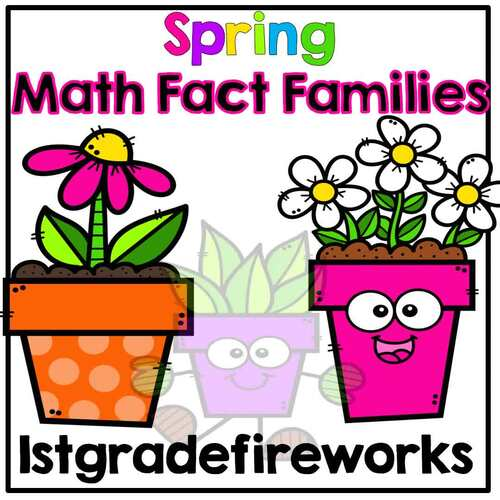# Spring Math Fact Family Booklets;
K - 2nd
Subjects
Resource Type
Standards
Formats Included
• PDF
Pages
21 pages
\$3.00
List Price:
\$5.00
You Save:
\$2.00
\$3.00
List Price:
\$5.00
You Save:
\$2.00
Report this resource to TPT

### Description

Helping students practice math facts to 20? ME too!

Spring Math Facts Booklets for student addition and subtraction practice.

• 21 math activity pages for students to use math mountains and number bonds to create addition and subtraction fact families.
• Student booklets in color and black and white ( students will LOVE to color when they are done!)
• Use with math manipulatives ( not included ), if need.
Total Pages
21 pages
N/A
Teaching Duration
N/A
Report this resource to TPT
Reported resources will be reviewed by our team. Report this resource to let us know if this resource violates TPT’s content guidelines.

### Standards

to see state-specific standards (only available in the US).
Apply properties of operations as strategies to add and subtract. If 8 + 3 = 11 is known, then 3 + 8 = 11 is also known. (Commutative property of addition.) To add 2 + 6 + 4, the second two numbers can be added to make a ten, so 2 + 6 + 4 = 2 + 10 = 12. (Associative property of addition.)
Add and subtract within 20, demonstrating fluency for addition and subtraction within 10. Use strategies such as counting on; making ten (e.g., 8 + 6 = 8 + 2 + 4 = 10 + 4 = 14); decomposing a number leading to a ten (e.g., 13 - 4 = 13 - 3 - 1 = 10 - 1 = 9); using the relationship between addition and subtraction (e.g., knowing that 8 + 4 = 12, one knows 12 - 8 = 4); and creating equivalent but easier or known sums (e.g., adding 6 + 7 by creating the known equivalent 6 + 6 + 1 = 12 + 1 = 13).